# Roof

Tiles are stacked in rows on the trapezoidal shaped roof.
At the ridge is 15 tiles and each subsequent row has one more tile than in the previous row.

How many tiled is covered roof if lowermost row has 37 tiles?

x =  598

### Step-by-step explanation: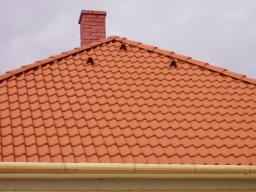Did you find an error or inaccuracy? Feel free to write us. Thank you!#### You need to know the following knowledge to solve this word math problem:

We encourage you to watch this tutorial video on this math problem:

## Related math problems and questions:

• Roof tilesThe roof has a trapezoidal shape with bases of 15 m and 10 m, height of roof is 4 meters. How many tiles will need if on 1 m2 should be used 8 tiles?
• Cinema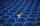How many rows of seats are in the stands, if you know that the first row has 24 seats and the last 50 seats and each subsequent row has two seats more than the row before? How many seats are in the stands?
• SeatsSeats in the sport hall are organized so that each subsequent row has five more seats. First has 10 seats. How many seats are: a) in the eighth row b) in the eighteenth row
• Cans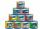How many cans must be put in the bottom row if we want 182 cans arrange in 13 rows above so that each subsequent row has always been one tin less? How many cans will be in the top row?
• Arithmetic progression 2The 3rd term of an Arithmetic progression is ten more than the first term, while the fifth term is 15 more than the second term. Find the sum of the 8th and 15th terms of the Arithmetic progression if the 7th term is seven times the first term.
• TheaterThe theater has in each row with 19 seats. Ticket to the first 10 rows is for 30 USD. In the next rows is for 15 USD. The theater was completely sold out. Revenue was 12255 USD. How many rows are in the theater?
• Students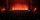In the front row sitting three students and in every other row 11 students more than the previous row. Determine how many students are in the room when the room is 9 lines, and determine how many students are in the seventh row.
• Chairs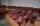Determine the number of seats in the seventh row and ninth row, if the 3rd row has 14 seats and in every next row of seats has five more than the previous row.
• Cinema 4In the cinema are 1656 seats and in the last row are 105 seats, in each next row 3 seats less. How many are the total rows in cinema?
• Salat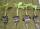Grandmother planted salad. In each row, he planted 13 seedlings. After a morning frost, many seedlings died. In the first row, five seedlings died. In the second row, two seedlings died more than 1st row. In the third row, three seedlings died less than 1
• Tiles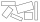The tile has the shape of a square with a side of 15 cm. What dimensions can a rectangle composed of 90 of these tiles have so that no tile remains?
• Cinema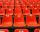Cinema auditorium is built for 3300 people. The first row is planned for 36 seats and each next gradually 4 more. How many rows of seats will have auditorium?
• Horses playgroundThe fence for the horses has the shape of a rectangular trapezoid with an area of 400 m2, the base lengths should be 31 m and 19 m. How many meters of boards will they need to fence it if the boards are stacked in 5 rows?
• Boxes200 boxes have been straightened in three rows. The first was 13 more than in the second, and in the second was one fifth more than in the third one. How many boxes are in each row?
• Tiles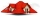The pupils of the building school have to calculate how many roof tiles they will need to cover the roof of the house in the shape of two rectangles measuring 10 x 7.2 m. One roof tile covers a rectangular area of 22 cmx32 cm. How many tiles will they nee
• Trees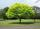Loggers wanted to seed more than 700 and less than 800 trees. If they seed in rows of 37, left them 8 trees. If they seed in rows of 43, left the 11 trees. How many trees must seed ?
• Dance ensembles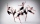4 dance ensembles were dancing at the festival. None had less than 10 and more than 20 members. All dancers from some of the two ensembles were represented in each dance. First, 31 participants were on the stage, then 32, 34, 35, 37, and 38. How many danc